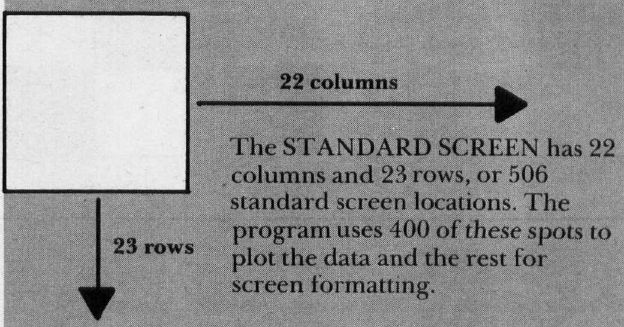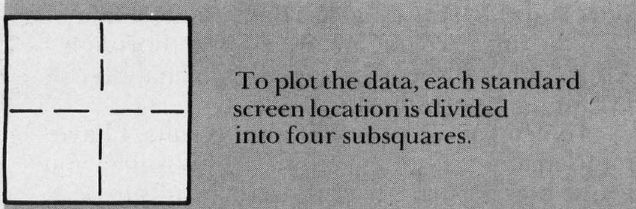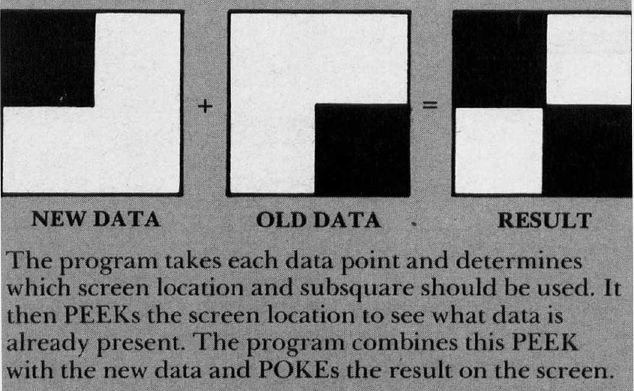`COMPUTE! ISSUE 30 / NOVEMBER 1982 / PAGE 223`

 VIC Plotting Matt UrnezisGarland, TX For information ranging from stock prices to the results of experiments, this program lets us easily analyze any information by displaying the data on a graph. It will also compute an equation to fit the data and then provide statistics to tell how good the fit is. We can display a medium resolution graph on the VIC by dividing each standard screen location into four equal subsquares. In this way, the 400 positions used in the program have the potential to represent 1,600 separate data points. The price that is paid to achieve the higher resolution is that data is no longer simply POKEd on the screen. The program first has to PEEK the screen location and combine it with the new data point to be displayed. This new combination is then POKEd back on the screen (see Figure 1). Besides graphing the data, the program will permit the user to statistically analyze the information by computing the equation for the straight line which best fits the data. Also listed is the coefficient of determination which tells how well the Y values are explained by the X values. This last item is the "standard error" of the estimate. This represents how far away the data points tend to be from the linear regression line. More thorough explanations of the meaning of each analysis can be found in most statistics books. In determining the statistical results, I have not used any rounding routines. This will let you decide how accurate you want the results to be. Unless you have a specific need, I would suggest adding the following four lines to make the information more readable.Figure 1A. ```515 B = INT (B * 1000 + .5)/1000 516 C = INT (C * 1000 + .5)/1000 525 R2 = INT (R2 * 1000 + .5)/1000 535 SD = INT (SD * 1000 + .5)/1000 ```Figure 1B.Figure 1C.